# [PL12] Derivations 2

So far you have learned two of the four quantifier rules. In this section you will learn the other two rules for the quantifiers.

## §1. Universal Quantifier Introduction

Universal Quantifier Introduction permits you, in some situations, to add a universal quantifier to a derivation. For example,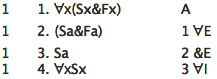This shows ∀x(Sx&Fx) ⊢ ∀xSx .

Here is a statement of ∀I:

#### ∀I (Universal Quantifier Introduction)

For any variable v and name c, if you have derived φv/c, and c does not occur in φ, and c does not occur in anything φv/c depends on, and ∀vφ is a well-formed formula of MPL, then you can write down ∀vφ, depending on everything φv/c depends on.

This may look a little complicated at first, but once you see the reason it is written that way, you will find it no more complicated than the quantifier rules you have learned already. Suppose you have written down φv/c in your derivation. If you want to write down ∀vφ using this rule you need to satisfy three restrictions. The first restriction is that c does not occur in φ. The second restriction is that c does not occur in anything φv/c depends on. The third and last restriction is that ∀vφ be a well-formed formula of MPL.

Let's think about why these restrictions are built into the rule.

If the third restriction was not present, then the following would be a correct derivation: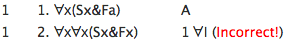The expression on line 2 is not a well-formed formula of MPL. One of the goals of our system is to make sure that each of the rules of our system is sound, that is, at each line in our derivation you write down a formula which is entailed by its dependencies. If you could write down line 2 we would have failed to achieve one of the goals of our system.

The second restriction on Rule ∀I is that the name c does not occur in anything φv/c depends on. If that restriction was not present, Rule ∀I would not be a sound rule. It is easy to see why: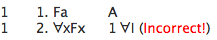"Fa" does not entail "∀xFx". So the formula on line 2 is not entailed by its dependencies. If you could use Rule ∀I to write down line 2, then Rule ∀I would not be sound. Rule ∀I does not permit you use line 1 to write down line 2 because "a" occurs in "Fa" and the "Fa" on line 1 depends on "Fa".

Explain why "Fa" does not entail "∀xFx".

The final restriction on Rule ∀I is that c does not occur in φ. If this restriction was not present then, again, Rule ∀I would not be a sound rule: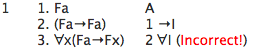In this example, φ is "(Fa→Fx)", v is "x", c is "a", and φv/c is "(Fa→Fa)". If Rule ∀I worked this way, we would be able to show ⊢ ∀x(Fa→Fx) even though it is not the case that ⊨ ∀x(Fa→Fx). Thus, if Rule ∀I worked this way, it would be unsound.

Explain why line 3 in the last example violates Rule ∀I.

Show that it is not the case that ⊨ ∀x(Fa→Fx).

## §2. Existential Quantifier Elimination

Now for the last quantifier rule, existential quantifier elimination. Here is an example: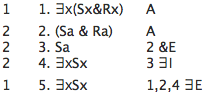Rule ∃E permits you to derive things when you have an existentially quantified formula as in "∃x(Sx&Rx)" on line 1. To use the rule, you assume an instance of the existentially quantified formula and then derive something from it. In this case "(Sa & Ra)" is assumed and "∃xSx" is derived from it. At this point Rule ∃E lets you write down what you have just derived a second time, changing its dependencies. While line 4 depended on the assumption in line 2, the rule lets you write down the same formula depending on the existentially quantified formula in line 1.

In essence, the idea behind the rule is this: when an existentially quantified formula like "∃xFx" is true under some interpretation, then you know that at least one formula like "Fa" or "Fb" or "Fc" or (and so on) is also true. But you don't know which one. Still, what you can do is assume one of these formulas (such as "Fb") and try to show something that you could show whether you assume "Fa" or "Fb" or "Fc" or whatever. For example, in the derivation above, we assumed "(Sa & Ra)" to derive "∃xSx". But we could have just as well assumed "(Sb & Rb)" or "(Sc & Rc)" etc. The choice of the name didn't matter in deriving "∃xSx". That shows that "∃xSx" follows not only from "(Sa & Ra)", but from "∃x(Sx&Rx)" as well.

Here is the rule, with all its restrictions:

#### ∃E (Existential Quantifier Elimination)

For any variable v and name c, if you have derived ∃vφ, assumed φv/c, and derived ψ, and c does not occur in ψ, φ, or anything ψ depends on (except φv/c), then you can write down ψ a second time, depending on everything the first ψ depends on (except the assumption φv/c) together with everything ∃vφ depends on.

Here are two examples of misuse of the rule, to help see how to use it correctly.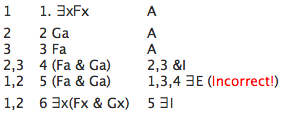In this last example, φ is "Fx", v is "x", c is "a", ∃vφ is "∃xFx", φv/c is "Fa", and ψ is "(Fa & Ga)". The problem here is that c occurs in ψ on line 4 ("a" occurs in the formula on line 4). So Rule ∃E cannot be used this way at line 5.

Here is another example of an incorrect use of the rule: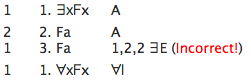To be sure, this example is not correct, otherwise you would be able to show that if something is fat, then everything is fat!

What restriction of the rule is violated on line 3 of the last example?